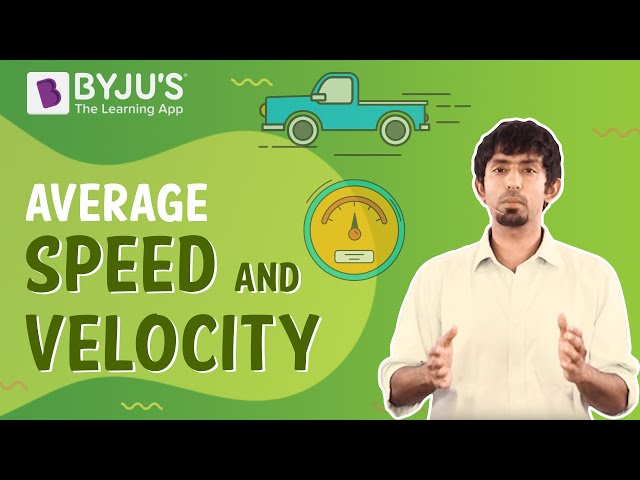Checkout JEE MAINS 2022 Question Paper Analysis : Checkout JEE MAINS 2022 Question Paper Analysis :

# Relation Between Torque And Speed

Torque is the rotational equivalence of linear force. Speed measures the distance covered in unit time. The relation between torque and speed are inversely proportional to each other. The torque of a rotating object can be mathematically written as the ratio of power and angular velocity.

### Torque and Speed Formula

 $$\begin{array}{l}Torque =\frac{Power}{speed}\end{array}$$ Or $$\begin{array}{l}\tau =\frac{P}{\omega }\end{array}$$

Where,

• P is the power (work done per unit time)
• τ is the torque (rotational ability of a body)
• ω is the angular speed/velocity (rate of change of angular displacement)

The above equation can be rearranged to compute the angular velocity required to achieve given torque and power. The torque injects power and it purely depends on instantaneous velocity.

## Connection Between Torque And Speed

For any rotatory motion, to derive the relation between torque and power, compare the linear equivalent. The linear displacement is the distance covered at the circumference of the rotation and is given by the product of angle covered and radius. Linear distance is given by the product of linear velocity and time.

=> Linear distance = radius × angular velocity × time

Torque makes object undergo rotational motion. It is expressed as-

=>

$$\begin{array}{l}Force=\frac{Torque}{Radius}\end{array}$$

Thus,

$$\begin{array}{l}Power=\frac{Force\times Linear\;distance}{Time}\end{array}$$

$$\begin{array}{l}Power=\frac{\left ( \frac{Torque}{Radius} \right )\times Radius \times Angular \;velocity \times Time}{Time}\end{array}$$

$$\begin{array}{l}Power=Torque\times Angular\;velocity\end{array}$$

$$\begin{array}{l}Torque =\frac{Power}{Angular\;velocity}\end{array}$$

## Motor torque Calculation

The rotational speed in motors is measured using rotations per minute. Thus on one rotation distance and linear speed is increases proportionally by 2??.

$$\begin{array}{l}Torque =\frac{Power}{2\pi \times Rotational\;speed}\end{array}$$

$$\begin{array}{l}Torque = \frac{Power}{2\pi \times Rotational\;speed}\times \frac{ft.lbf}{min}\times \frac{hp}{33000\left ( \frac{ft.lbf}{min} \right )}\end{array}$$

$$\begin{array}{l}\approx \frac{torque\times RPM}{5252}\end{array}$$

Where,

$$\begin{array}{l}\frac{33000}{2\pi }\approx 5252.113122\end{array}$$

Hope you understood the relation and conversion between the Power and the Speed of a rotating object.

Physics Related Topics:

Stay tuned with BYJU’S for more such interesting articles. Also, register to “BYJU’S – The Learning App” for loads of interactive, engaging physics related videos and an unlimited academic assist.

## Learn how to calculate average speed and average velocity easily.## Frequently Asked Questions – FAQs

### What is the formula for torque?

The formula for torque is Torque = Power/Speed

True.

### What does angular speed define?

Angular speed defines how fast the central angle of a rotating body changes with respect to time.

### What is linear displacement?.

Distance covered at the circumference of the rotation gives the linear displacement. It is given by the product of angle covered and radius.

### What is the formula to calculate linear distance?

Linear distance = radius × angular velocity × time

Test Your Knowledge On Relation Between Torque And Speed!